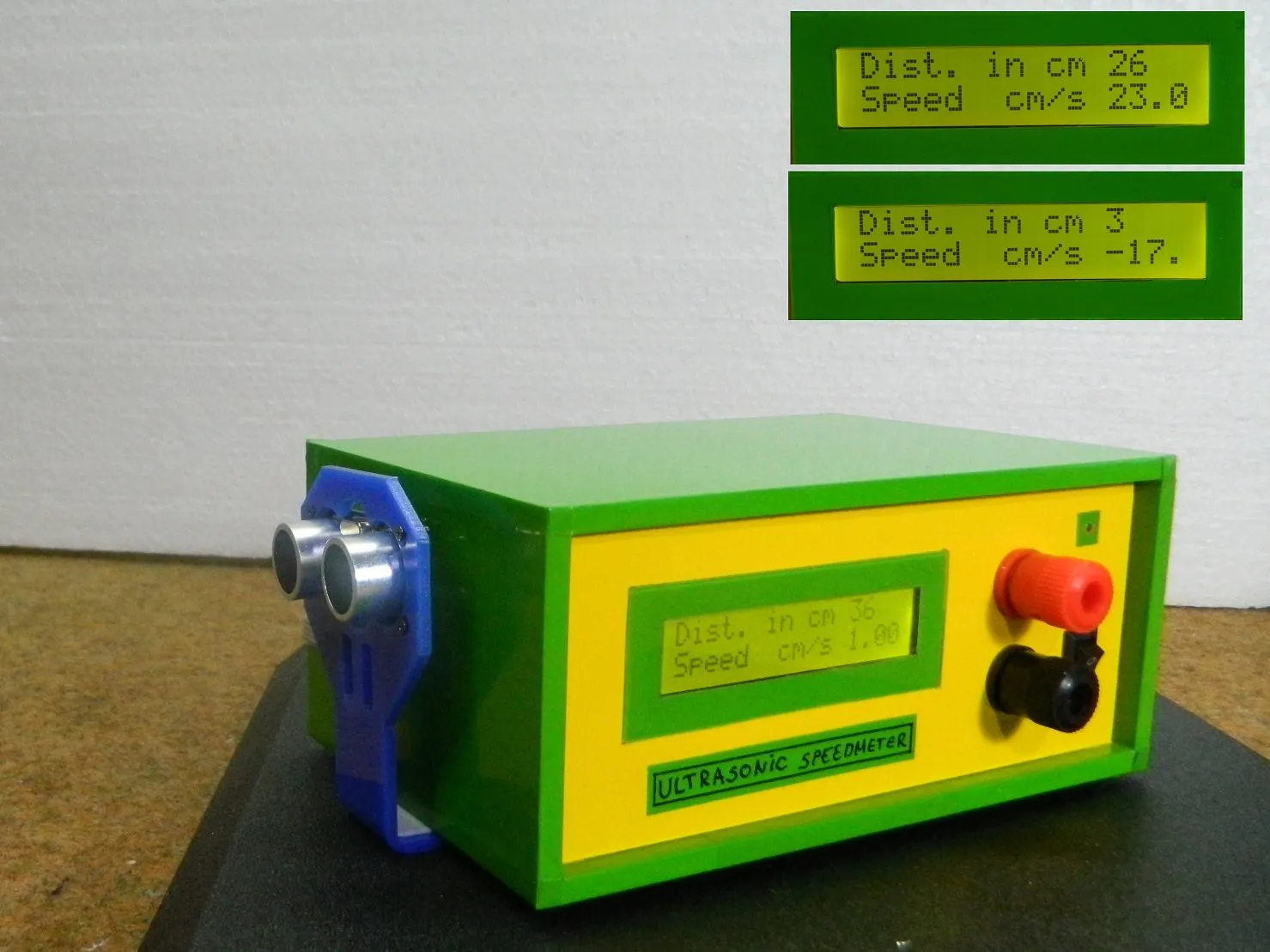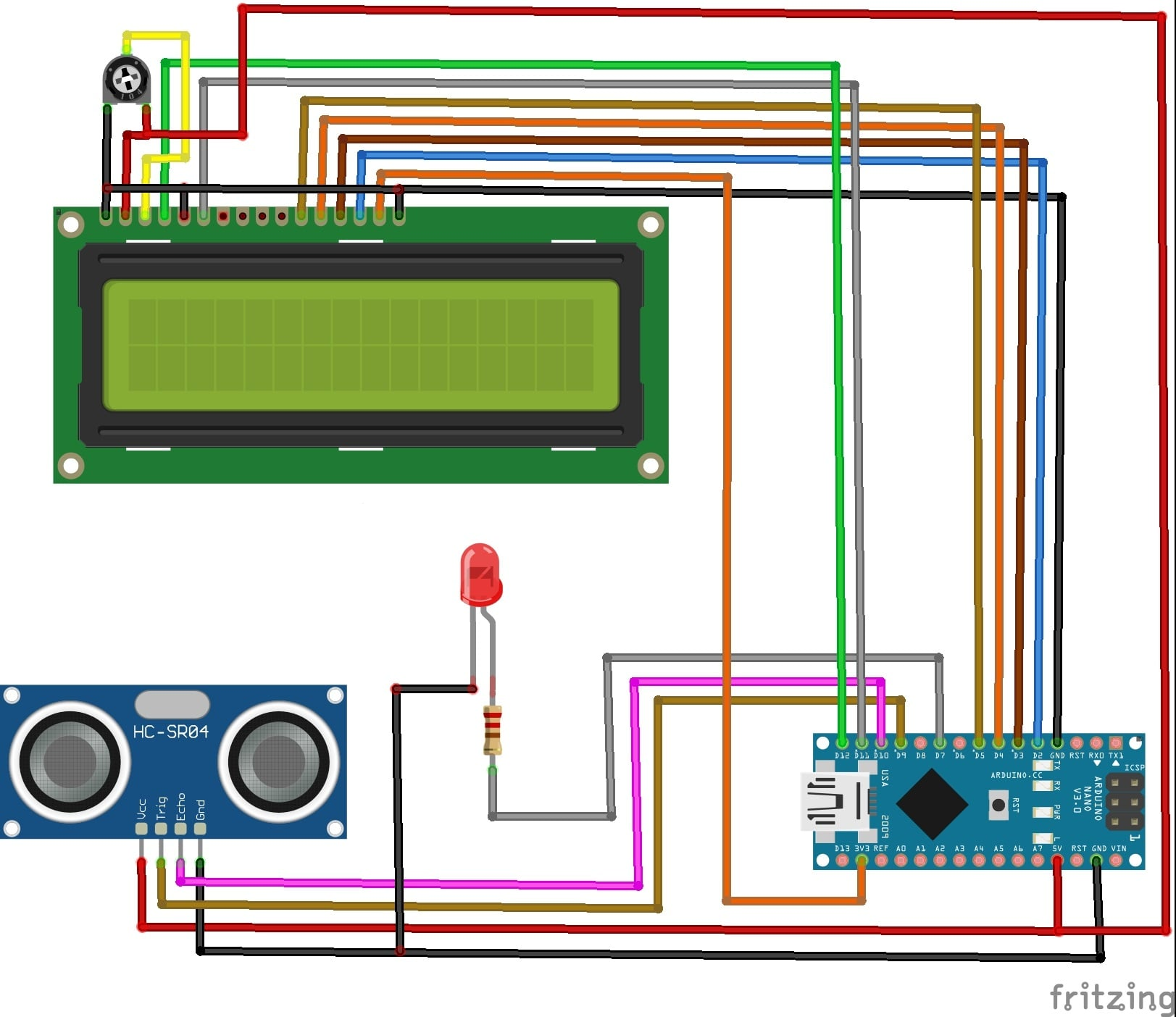Anduino

# Speed Measurement Using HC-SR04 Ultrasonic SensorMay 08, 2021 by Mirko Pavleski
ShareOn this project I will show you how to measure the speed of movement of an object using HC-SR04 ultrasonic sensor.

## Hardware

 1 Arduino Nano R3 1 Adafruit Standard LCD - 16x2 White on Blue 1 Ultrasonic Sensor - HC-SR04 (Generic) 1 LED (generic) 1 Resistor 330 ohm 1 Single Turn Potentiometer- 10k ohms

## Software

 1 Arduino IDE

## Tools

 1 Soldering iron (generic) 1 Solder Wire, Lead Free

HC-SR 04 Ultrasonic sensor is most commonly used to measure distance, but this time I will show you how to measure the speed of movement of an object using this sensor.

Device is very simple and consist only a few components:

- Arduino Nano microcontroller

- LCD display

- Ultrasonic sensor

- and LED diode

For that purpose we need to take two distance measurements in a short time apart and we have:

distance2 - distance1 = distance speed at a given time

If we make the measurements in a time period of 1 second, then we get the speed of movement of the object in cm/s. The basic code is taken from the arduino cc forum and I just added an LCD display for a visual representation of the results.

The first row shows the distance, and the second row shows the speed if the object is moving.

There is also an LED that signals the distance at which the object is placed. If the distance is less than 5 cm, the LED lights up continuously. As the distance increases the LED starts flashing at a speed that depends on the distance of the object. If the object moves away, the blinking is slower, and vice versa.

When the object is moving in the opposite direction, the speed represented on the display has a negative sign.

``````#include <LiquidCrystal.h>
LiquidCrystal lcd(12, 11, 5, 4, 3, 2);// RS,E,D4,D5,D6,D7
// defines pins numbers
const int trigPin = 9;
const int echoPin = 10;

// defines variables
long duration;
int distance1=0;
int distance2=0;
double Speed=0;
int distance=0;

void setup()
{
lcd.begin(16, 2);// LCD 16X2
pinMode(trigPin, OUTPUT); // Sets the trigPin as an Output
pinMode(echoPin, INPUT); // Sets the echoPin as an Input
pinMode( 7 , OUTPUT);
Serial.begin(9600); // Starts the serial communication
}

void loop()
{

//calculating Speed

delay(1000);//giving a time gap of 1 sec

//formula change in distance divided by change in time
Speed = (distance2 - distance1)/1.0; //as the time gap is 1 sec we divide it by 1.

//Displaying Speed
Serial.print("Speed in cm/s  :");
Serial.println(Speed);
lcd.setCursor(0,1);
lcd.print("Speed  cm/s ");
lcd.print(Speed);

// LED indicator
if (distance >0 && distance <5)
{
digitalWrite( 7 , HIGH);
delay(50);                  // waits for a second
}

if (distance > 5 && distance <10 )
{
digitalWrite( 7 , HIGH);
delay(50);                  // waits for a second
digitalWrite( 7 , LOW);    // sets the LED off
delay(50);                  // waits for a second
}

if (distance >10  && distance < 20)
{
digitalWrite( 7 , HIGH);
delay(210);                  // waits for a second
digitalWrite( 7 , LOW);    // sets the LED off
delay(210);                  // waits for a second
}

if (distance >20  && distance < 35)
{
digitalWrite( 7 , HIGH);
delay(610);                  // waits for a second
digitalWrite( 7 , LOW);    // sets the LED off
delay(610);                  // waits for a second
}

}

{
// Clears the trigPin
digitalWrite(trigPin, LOW);
delayMicroseconds(2);

// Sets the trigPin on HIGH state for 10 micro seconds
digitalWrite(trigPin, HIGH);
delayMicroseconds(10);
digitalWrite(trigPin, LOW);

// Reads the echoPin, returns the sound wave travel time in microseconds
duration = pulseIn(echoPin, HIGH);

//calculating distance
distance= duration*0.034/2;

// Prints the distance on the Serial Monitor
Serial.print("Distance in cm : ");
Serial.println(distance);
lcd.setCursor(0,0);
lcd.print("Dist. in cm ");
lcd.print(distance);
lcd.print("   ");
return distance;

}``````